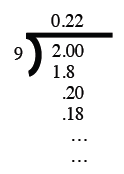### Home > MC2 > Chapter 2 > Lesson 2.1.1 > Problem2-11

2-11.

Rewrite the following fractions as decimals and decide if they are terminating or repeating decimals. Show your work and explain how you made your decision.

1. $\frac { 7 } { 8 }$

Divide $7$ by $8$. Do you get a repeating decimal?

Since $7$ divided by $8$ is $0.875$, and this does not repeat without end, it is a terminating decimal.

1. $\frac { 2 } { 9 }$

Here is Kelly's work.Does it look like the decimal will repeat?

1. $\frac { 4 } { 7 }$

See parts (a) and (b).

Repeating.

1. $\frac { 11 } { 22 }$

See parts (a) and (b).